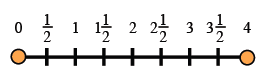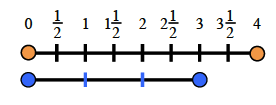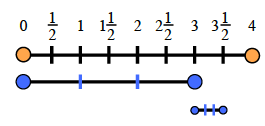### Home > MC1 > Chapter 6 > Lesson 6.2.5 > Problem6-93

6-93.

. Jill lives 3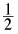miles from school. One morning, her friend was giving her a ride. When they were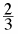of the way to school, their car broke down and they had to walk the rest of the way. Draw a picture to help you figure out how far they walked. Homework Help ✎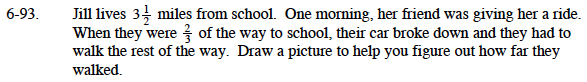$\text{If Jill and her friend were able to drive }\frac{2}{3}\text{ of the way to school, then they had to walk }\frac{1}{3}\text{ of the way.}$

$\text{Can you find what } \frac{1}{3}\text{ of }3\frac{1}{2}\text{ is?}$

$\text{The number line below is numbered 0 through 4. In order to find the total value of }\frac{1}{3}\text{ of } 3\frac{1}{2},$

$\text{ it will help to split up the problem.}$

$\text{First, find } \frac{1}{3}\text{ of } 3.$

$\text{Now find } \frac{1}{3}\text{ of } \frac{1}{2}.$

Were you able to find the distance Jill walked?
Remember to draw a picture of your own!